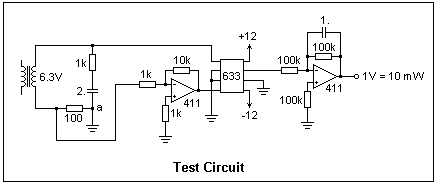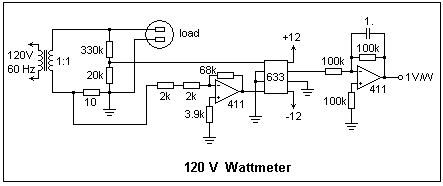## Wattmeter

Building a wattmeter using the AD633 analog multiplier

### Electrical Power

Electrical power in watt or joule/s delivered to a device is the product of the voltage between the input terminals and the current entering at the positive input terminal, p = vi. If this product is negative, then the device is delivering energy to the external circuit. In a DC circuit under steady conditions v and i are constant, and so is the power. Therefore, power can be determined by simultaneous measurements of current and voltage.

In an AC circuit with sinusoidal current and voltage, these quantities are constantly changing, and so then is the power. The voltage and current measured by instruments are only representative values, usually the rms or effective values, which are the peak values of the sinusoids divided by the square root of two. The power averaged over a period is no longer simply VI, where V and I are the rms values, but P = VI cos φ, where φ is the phase difference between the voltage and current waves. The power factor is cos φ. In an inductive circuit, the voltage leads the current, while in a capacitive circuit, the current leads the voltage. If the phase differences are the same, the power factor has the same sign in both cases, so a power factor is usually quoted as lagging (inductive) or leading (capacitive), where lagging or leading refers to the relation of the current wave to the voltage wave.

If we express the voltage and current as phasors, v = v(t) = Vejωt and i = i(t) = Iejωt - φ, the instantaneous power must be written as the product of the real parts of these two expressions, which are V cos ωt and I cos(ωt - φ). The final result, after using the usual trigonometric identites, is p(t) = (VI/2)cos φ + (VI/2)cos(2ωt - φ). The first term is a constant, while the second term is a sinusoidal variation of double frequency. The average power is simply given by the constant term, or P = (VI/2)cos φ, which is just the product of the rms values times the power factor as defined above.

In terms of the phasors, we also see that P = Re(vi*/2) = Re(v*i/2). Note that the complex conjugate of one factor appears, there is a division by 2, and the real part is taken.

We cannot measure AC power using simultaneous measurements of rms values of current and voltage. A special instrument is necessary that takes into account the phase difference, which is called a wattmeter. Although wattmeters were once a common AC lab instrument, they are not found in current catalogs of electronics suppliers. You might want one for testing transformers, for example. It is not difficult to build your own, however!

### The Wattmeter

The traditional wattmeter was an electrodynamometer instrument with a rotating potential coil of many turns and a fixed current coil of a small number of turns. The torque on the moving coil was proportional to the product of current and voltage, and therefore to the instantaneous power. The inertia of the moving coil and pointer averaged the torque. Multipliers and shunts could be used as with ordinary voltmeters and ammeters. The scale had to be calibrated, since the torque depended on the coil position.

A wattmeter can also be made by multiplying signals proportional to the voltage and current, using an AD633 analog multiplier, and averaging the result with an op-amp integrator. The signals applied to the 633 should be in the range ±10 V. If these are V' = AV and V" = BI, where V is in volts and I is in amperes, then the output of the 633 will be V'V"/10 = (AB/10)VI, so that AB/10 volts output corresponds to 1 W. The AD633 is very easy to use, and is ideal for this application. Use a bipolar power supply of at least ±12V (maximum ±18V).A test circuit is shown at the right, which is easily assembled on a solderless breadboard. The load to which the power is to be measured is represented by the 1k resistor in series with a 2 μF capacitor (which cannot be electrolytic, of course). The voltage is applied by a transformer. Since here the voltage is applied directly to the 633, about 6.3 V rms is the maximum that is allowed. In most cases, a voltage divider would be used so that the voltage applied to the 633 is less than ±10 V. The transformer is necessary, especially if the AC supply is the mains supply, for safety as well as so node "a" on one side of the load can be assumed to be ground. The current sensing resistor is chosen as 100 Ω, and the voltage is amplified by a factor 10 by the inverting amplifier using an LF411. In general, the sensing resistor and the gain of the amplifier can be chosen as convenient in any particular case.

For this circuit, A = 1 and B = 100 x 10 I, so 1 W corresponds to 100 V, or 1 V output corresponds to 10 mW dissipated in the load. Note that the current factor is connected so that a positive output voltage will correspond to a positive power dissipation.

The averaging amplifier is an integrator with a low-frequency gain of unity, and a time constant of 0.1 s, which is satisfactory for 60 Hz. The resistors connected to the noninverting inputs of the op-amps reduce the effects of bias currents.

On test, the voltage across the load was measured by a voltmeter, from which the power dissipated in the resistor can be calculated by the usual circuit theory. This quantity was compared with the voltage output from the circuit, and the agreement was very good. The wattmeter does not have to be calibrated, if normal component tolerances are acceptable. The power factor in this case is 0.60 leading. The load current could also be easily measured, which would allow this power factor to be found experimentally, as P/VI.

It is instructive to look at the signals with an oscilloscope, and to estimate the phase angle independently (it is -53° for the test load). The output of the 633 is also interesting, where the frequency doubling is clearly seen.

The circuit can also be tested with direct current. Replace the load with, say, a 4.7k resistor, and apply 8-10 V. Compare the output voltage with the calculated power. I used a 4.7k load, and 7.66 V, measuring 12.70 mW, which is 1.8% high. The circuit works for any higher frequency than 60 Hz as well as at DC. For a lower frequency the time constant of the integrator has to be increased. Furthermore, the accuracy of the circuit does not depend on the waveform; the average power is measured for any waveform.

### A Practical WattmeterThe modifications required to build a practical 120V wattmeter are shown at the left. With the 10Ω current sensing resistor, the meter reads to 10W; if a 1Ω resistor is used instead, the range is increased to 100W. It is easy to include meters reading the voltage across the load and the load current, so one can get P, V and I. 1% resistors can be used in place of the 5% resistors if a more precise calibration is necessary. The divider resistors can be 1/4W resistors. The current sensing resistor should be 5 W for currents up to 0.7A rms, or even larger if larger load currents are expected. In any case, make certain that the resistors can dissipate the required power, and peak voltages to the 633 do not exceed 10V. The circuit can be fed with a Variac to set the load voltage as desired. Of course, this wattmeter works at any voltage less than 120V with no change. Again, it should be emphasized that the isolation transformer must not be omitted.

The power indicated by this meter includes the power dissipated in the voltage divider, which is easily found once the load voltage is known. For 120V, this is 41 mW, which should be subtracted from the indicated power. This can be measured in the absence of a load other than the voltage divider. Care should be taken that the meter is not overloaded (voltage inputs to the AD633 exceeded) at low power factors, when the output voltage may be small, while the currents and voltages are not. It is a peculiarity of wattmeters that they can be seriously overloaded while still reading low. Remember that the peak voltage of 120V AC is 170V. Test the wattmeter at DC to verify the calibration.

### References

The AD633 is discussed at length in Experiment 30.

Short-circuit and open-circuit tests of transformers are explained in any text on AC machinery. Usually, the primary is excited with the secondary shorted, and the secondary is excited with the primary open, respectively (for a step-down transformer). A Variac is used since the voltage applied is low in either case. In the open-circuit test, the wattmeter measures the core loss.

Return to Electronics Index

Composed by J. B. Calvert
Created 13 July 2008
Last revised